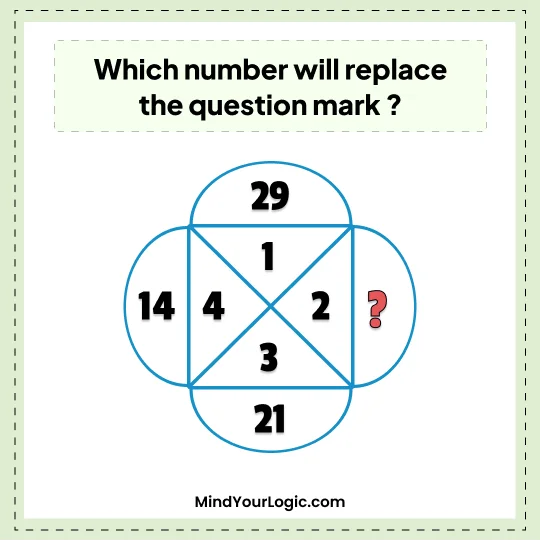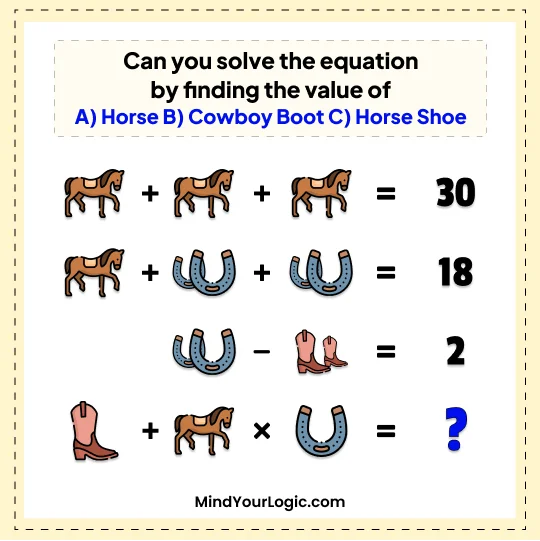# 100+ Challenging math riddles to keep your mind sharp#### A quarter is one fourth of a dollar - Math Riddle

###### 121.Math Riddles
```A quarter is one fourth of a dollar but Mr. Brainteaser has changed the equation as below :
DOLLAR * 4 = QUARTER
Can you replace each letter by a digit to make above solution correct ?```
`1830920`
```Explanation :

DOLLAR * 4 = QUARTER
457730 * 4 = 1830920```

#### Replace the question mark with number - Math Riddle

###### 122.Math Riddles
`Which number will replace the question mark ?`• A.26
• B.30
• C.25
• D.45
A.26
```Explanation :

26
14 = 1^2 + 2^2 + 3^2
21 = 4^2 + 1^2 + 2^2
29 = 4^2 + 3^2 + 2^2
26 = 3^2 + 4^2 + 1^2```

#### There is a unique number - Math Riddle

###### 123.Math Riddles
```There is a unique number which when multiplied by any number from 1 to 6, we will get the new number that contains same digits only.
Can you find that number ?```
`142857`
```Explanation :

142857
142857 * 1 = 142857
142857 * 2 = 285714
142857 * 3 = 428571
142857 * 4 = 571428
142857 * 5 = 714285
142857 * 6 = 857142
These number are considered as cyclic numbers.```

#### For long they are racing ? - Math Riddle

###### 124.Math Riddles
```Before the start of the car race, Tony Stewart and Jamie Mcmurray have the same amount of fuel in the car. With this fuel Tony Stewart can drive for 4 hours while Jamie can drive five hours. After a time they realize that amount of
fuel left in Tony car is 1/4th of the fuel in Jamie car. For long they are racing ?```
`5/6 hours`
```Explanation :

Let's call the amount of fuel in each car as "x".

Tony Stewart's car can drive for 4 hours with x amount of fuel, so his fuel consumption rate is x/4.
Jamie Mcmurray's car can drive for 5 hours with x amount of fuel, so his fuel consumption rate is x/5.

After a certain amount of time, they realize that the amount of fuel in Tony's car is 1/4th of the fuel in Jamie's car. So, x/4 = x/5 * 1/4.

Solving for x, we get x = 20.

So the amount of fuel in each car is 20.

Now, we can use the fuel consumption rate of each car to find out how long they have been racing.

Let's call the time they have been racing as "t".

Tony's car has x - x/4 * t = 20 - 20/4 * t = 20 - 5t amount of fuel left.
And Jamie's car has x - x/5 * t = 20 - 20/5 * t = 20 - 4t amount of fuel left.

We know that Tony's car has 1/4th of the fuel left in Jamie's car, so:
20 - 5t = (1/4) * (20 - 4t)
Expanding and simplifying, we get:
20 - 5t = 5 - t
25 - 6t = 5
t = 5/6 hours.

So, they have been racing for 5/6 hours.```

#### Horse Cowboy Boot and Horseshoe Viral - Math Riddle

###### 125.Math Riddles
```Can you solve the equation by finding the value of
A) Horse
B) Cowboy Boot
C) Horse Shoe````Horse = 10, Cowboy Boot = 1 ,Horse Shoe= 2`
```Explanation :

Horse = 10, Cowboy Boot = 1 ,Horse Shoe= 2

= Step 1 =
3 Horse = 30
= Horse = 10 .....(A)

= Step 2 =
1 Horse + 2 Horse Shoe + 2 Horse Shoe = 18
10 + 4 Horse Shoe =18
HorseShoe = 2 .....(C)

= Step 3 =
2 Horse Shoe - 2 Cowboy Boot = 2
4 - 2 Cowboy Boot = 2
= Cowboy Boot = 1 ... (B)

= Step4 =
Cowboy Boot + Horse * Horse Shoe = 1+10*2 = 21 ```

#### Lena Dunham , Allison Williams, and Jemima Kirke - Math Riddle

###### 126.Math Riddles
```Lena Dunham, Allison Williams, and Jemima Kirke are 3 daughters of a good mathematician "Geroge". When I asked George the age of their daughters. He replied "The current age of her daughters is prime. Also, the difference between their ages is also prime? How old are the daughters?
```

```Explanation :

Lena Dunham : 2 , Allison Williams : 5 and Jemima Kirke : 7
Age diff : 7-2 is 5 , 7-5 is 2
and 5-2 is 3 which all are prime numbers```

#### Equation Math Puzzle

###### 127.Math Riddles
`1000 + 40 + 1000 + 30 + 1000 + 20 + 1000 + 10 = ?`
• A.5000
• B.4100
• C.3500
• D.6000
B.4100
```Explanation :

4100.
1000 + 40 = 1040
1040 + 1000 = 2040
2040 + 30 = 2070
2070 + 1000 = 3070
3070 + 20 = 3090
3090 + 1000 = 4090
4090 + 10 = 4100```